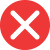equasion

Incorrect spelling, explanation: suffixes -sion and -tion are often confused, but there are some rules which can help us to distinguish them. One such rule is, that when the verb ends with the suffix -ate, then its noun form ends with -tion. This is exactly what happens with equation: the verb form is to equate, so we should use the suffix -tion. This also explains why equasion is incorrect.equation

Correct spelling, explanation: equation is the correct spelling because the verb to equate points to -tion suffix. This is due to the fact, that there are two suffixes that are often confused: -sion and -tion. According to one of the rules verbs that end with -ate (as in equate) end with -tion when transformed into nouns. Therefore equation is the correct form and equasion is incorrect.

Definition of equation:
noun, in mathematics: a formula in which two expressions are equal; also: a complicated situation
Another important factor in the equation is the interest rate.
In equation 3x – 2 = 4, x equals 2.

Collocations with equation:
basic / simple equation
The formula for finding the volume of a sphere is a basic equation in geometry.
complex / complicated equation
The Navier-Stokes equations describe fluid dynamics and can be a complicated equation to solve.
solve / work our an equation
In order to find the value of x, you need to solve an equation.
equation for
The equation for calculating the force of gravity between two objects is a fundamental concept in physics.
come into an equation
Environmental factors such as temperature and humidity come into an equation when calculating air pressure.equetion

Incorrect spellingequaton

Incorrect spelling